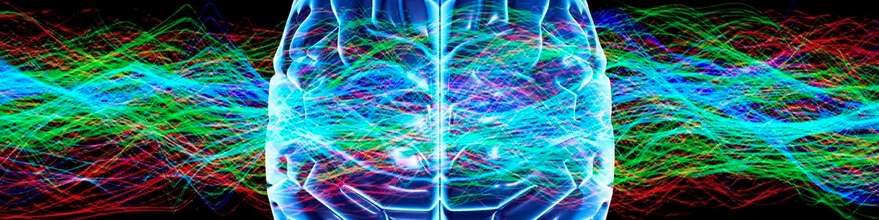# PyTorch: Times Series Classify Binary

Binary Detection of Epileptic Seizures Using a Cohort of Sequence of Electroencephalography (EEG) Readings.Sequence data structures contain many observations (rows) for each sample (e.g. site, sensor, or patient). They are often used for grouping time-based observations into what is called a time series. However, sequences can also represent biological sequences like DNA and RNA.

The cardinality of many observations per sample changes the dimensionality of the data from 2D to 3D. This effectively adds an additional layer of complexity to all aspects of data preparation. In this notebook, you’ll see that, once a Dataset.Sequence has been ingested, the AIQC API allows you to work with multivariate 3D data as easily as if it were 2D. As an example, you can still apply encoders by dtype and column_name.

## 💾 Data

This dataset is comprised of:

• Features = a sequence of electroencephalogram (EEG) readings.

• Label = presence of an epileptic seizure.

:

from aiqc import datum
df = datum.to_df('epilepsy.parquet')
df.sample(5)

:

sensor_0 sensor_1 sensor_2 sensor_3 sensor_4 sensor_5 sensor_6 sensor_7 sensor_8 sensor_9 ... sensor_169 sensor_170 sensor_171 sensor_172 sensor_173 sensor_174 sensor_175 sensor_176 sensor_177 seizure
0 232 183 125 47 -32 -73 -105 -99 -72 -33 ... -202 -303 -365 -389 -406 -401 -366 -251 -143 1
1 284 276 268 261 254 241 232 223 212 206 ... 64 15 -19 -57 -91 -118 -131 -140 -148 1
2 373 555 580 548 502 433 348 276 216 182 ... -1032 -1108 -803 -377 -13 172 246 206 156 1
3 791 703 538 76 -535 -1065 -1297 -1018 -525 -13 ... -396 135 493 601 559 400 193 3 -141 1
4 436 473 508 546 587 615 623 615 596 574 ... 637 644 646 650 656 653 648 628 608 1

5 rows × 179 columns

:

from aiqc.orm import Dataset

:

label_df = df[['seizure']]
label_dataset = Dataset.Tabular.from_df(label_df)

[ ]:

seq_3D = df.drop(columns=['seizure']).to_numpy().reshape(1000,178,1)
feature_dataset = Dataset.Sequence.from_numpy(arr3D_or_npyPath=seq_3D)


## 🚰 Pipeline

:

from aiqc.mlops import Pipeline, Input, Target, Stratifier
from sklearn.preprocessing import StandardScaler, LabelBinarizer

:

pipeline = Pipeline(
Input(
dataset  = feature_dataset,
encoders = Input.Encoder(StandardScaler(), dtypes=['int64'])
),

Target(
dataset = label_dataset,
column  = 'seizure',
encoder = Target.Encoder(LabelBinarizer())
),

Stratifier(
size_test       = 0.12,
size_validation = 0.22
)
)


└── Info - System overriding user input to set sklearn_preprocess.copy=False.
This saves memory when concatenating the output of many encoders.



## 🧪 Experiment

:

from aiqc.mlops import Experiment, Architecture, Trainer
import torch.nn as nn
import torchmetrics as tm
from aiqc.utils.pytorch import fit

:

def fn_build(features_shape, label_shape, **hp):
# LSTM() returns tuple of (tensor, (recurrent state))
class extract_tensor(nn.Module):
def forward(self,x):
# Output shape (batch, features, hidden)
tensor, _ = x
# Reshape shape (batch, hidden)
return tensor[:, -1, :]

model = nn.Sequential(
nn.LSTM(
input_size = features_shape,
hidden_size = hp['hidden'],
batch_first = True
),
extract_tensor(),
nn.Linear(hp['hidden'],1),
nn.Sigmoid(),
)
return model

:

def fn_train(
model, loser, optimizer,
train_features, train_label,
eval_features, eval_label,
**hp
):
model = fit(
model, loser, optimizer,
train_features, train_label,
eval_features, eval_label
, epochs     = hp['epochs']
, batch_size = hp['batch_size']
, metrics    = [tm.Accuracy(), tm.F1Score()]
)
return model

:

hyperparameters = dict(
hidden       = 
, batch_size = 
, epochs     = [5, 10]
)

:

experiment = Experiment(
Architecture(
library           = "pytorch"
, analysis_type   = "classification_binary"
, fn_build        = fn_build
, fn_train        = fn_train
, hyperparameters = hyperparameters
),

Trainer(pipeline=pipeline, repeat_count=1)
)

:

experiment.run_jobs()

📦 Caching Splits 📦: 100%|██████████████████████████████████████████| 3/3 [00:00<00:00, 220.57it/s]
🔮 Training Models 🔮: 100%|██████████████████████████████████████████| 2/2 [00:57<00:00, 28.78s/it]


## 📊 Visualization & Interpretation

For more information on visualization of performance metrics, reference the Dashboard documentation.